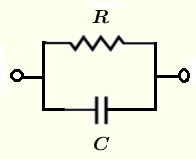# Parallel RC circuit Impedance Calculator

A calculator to calculate the equivalent impedance of a resistor and a capacitor in parallel. The calculator gives the impedance as a complex number in standard form and polar forms.

  

## Formulae for Parallel R C Circuit Impedance Used in the Calculator and their Units

We first give the formulas used in the parallel RC calculator and the proof of these formulas is presented in the bottom part of the page.Let $f$ be the frequency, in Hertz, of the source voltage supplying the circuit.
and define the following parameters used in the calculations
$\omega = 2 \pi f$ , angular frequency in rad/s

$X_C = 1 / (\omega C)$ , the capacitive reactance in ohms $(\Omega)$
The impedance of the capacitor $C$ is given by
$Z_C = \dfrac{1}{j \omega C} = -\dfrac{j}{\omega C}$

Let $Z$ be the equivalent impedance to the parallel RC circuit shown above and write it in complex form as follows
$\dfrac{1}{Z} = \dfrac{1}{R} + \dfrac{1}{Z_C}$
$Z = \dfrac{R Z_C} {R + Z_C} = \dfrac{R \dfrac{1}{j \omega C}}{R+\dfrac{1}{j \omega C}} = \dfrac{1}{j \omega C + \dfrac{1}{R}}$
The formulae for the modulus $|Z|$ and argument (or phase) $\theta$ of $Z$ are given by

Modulus: $|Z| = \dfrac{1}{\sqrt{ \omega^2 C^2 + \dfrac{1}{R^2} } }$ in ohms $(\Omega)$

Argument (Phase): $\theta = \arctan ( - R \omega C )$ in radians or degrees

## Use of the calculator

Enter the resistance, the capacitance and the frequency as positive real numbers with the given units then press "calculate".

 Resistance R = 50 mΩ Ω KΩ MΩ Capacitance C = 15 pF nF μF mF F Frequency f = 1.5 GHz MHz kH Hz mHz Number of Decimals 4